# Low pass filter (uniform/gauss distribution)

evol_w10lv

## Homework Statement

[/B]
I have got data x, which is formed like uniform distribution.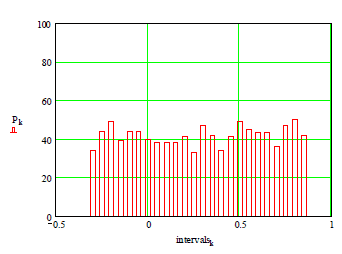After using discrete low pass filter I got output data u, which is Gauss distribution.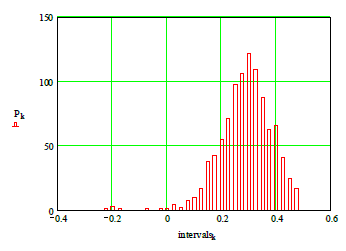What is explanation, why using that filter, we from uniform distribution can get Gauss distribution?

## Homework Equations

Low pass filter formula: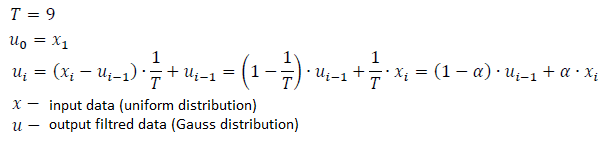## The Attempt at a Solution

[/B]
Filtred data starts to reach mean value of input data.

Homework Helper
Gold Member
Discrete time filtering of noisy signals essentially involves the addition of scaled, random numbers. Each input sample can be thought of being associated with a random number (conforming to a random variable with some known distribution, such as uniform distribution, for example).

Each output sample involves scaling these random numbers by some amount and adding them together. In an IIR filter (like this one), this also involves summing new, scaled inputs (new random numbers) together with scaled, previous outputs (old random numbers). The point of what I'm trying to say here is it involves the summing of random numbers.

-----

Let's take a step back a little and talk about the summing of random variables.

Flip a fair coin. It's either heads or tails. Let's assign 0 to tails and 1 to heads. It's uniformly distributed between 0 and 1.
0 [p = 1/2] tails

Now flip two coins (or the same coin twice) and add the results together. The distribution isn't uniform any more. The possible outcomes are:
0 [p = 1/4] (both tails)
2 [p = 1/4] (both heads)

Now flip the coin three times. Possible outcomes:
0 [p = 1/8] (all tails)
3 [p = 1/8] (all heads)

Four flips:
0 [p = 1/16] (TTTT)
1 [p = 4/16] (HTTT --or-- THTT --or-- TTHT --or-- TTTH)
2 [p = 6/16] (HHTT --or-- HTHT --or-- HTTH --or-- THHT --or-- THTH --or-- TTHH)
3 [p = 4/16] (HHHT --or-- HHTH --or-- HTHH --or-- THHH)
4 [p = 1/16] (HHHH)

Notice that as we sum together more random flips that the distribution function gets smoother and closer to a bell shape.

What would happen if we increased the number of flips to a very large number?

---

Now back to the discrete filter. Things might be a little more complicated here because the some of the random variables are scaled before they are added.

What does the Central Limit Theorem, or something very much like it, tailored to this situation, have to say about this?

•evol_w10lv
Homework Helper
Gold Member
I don't want you to get bogged down with the Central Limit Theorem. It does apply in the case of adding up all the coin flips. But I only mentioned it in my above post as a point of reference (and the fact that the Central Limit Theorem involves the Gaussian distribution function).

But it doesn't really apply directly in the case of the discrete filter because there is weighting and scaling going on. The distribution of the output of your discrete filter is not truly Guassian, even if it is pretty close.

If you wish to find the true, mathematically accurate probability distribution of the filter's output, it may help to study what happens when you add two random variables together, each with a different probability distribution, and calculate the distribution of the sum. (Hint: Discrete convolution is involved in the distribution function of the sum). [Edit: Actually that's continuous (not discrete) convolvution that is involved in the probability density function of the sum. The filter performs discrete convolution when its doing its filtering thing, but if we want to find solve for the probability density functions, continuous convolution is involved.]

Take that a step further and iteratively ["recursively" might be the better word] convolve the weighted, previous distribution function of the output with the weighted, distribution function of the input. The mathematics can get scary, but if you can do it, you'll have your precise answer.

I'm not sure if this is what you are looking for, or if you are trying to find a more subjective (less precise) answer.

[Edited for clarification.]

Last edited:
•evol_w10lv
evol_w10lv
I like your approach to this question. I hadn't thought, that output is sum of two different distributions. Actually output isn't Gaussian distribution, but it just looks like that?

Filter output can be calculated recursively using difference equation:
$$y(n) = \sum_{k=0}^M b_nx(n-k)+\sum_{k=1}^N a_ky(n-k)$$
Or using convolution operation:
$$y(n) =h(n) * x(n),$$
where ##h(n)## is impulse response.
In my case transfer function of a IIR discrete time filter:
$$H(z) =\frac {0.111} {1-0.889z^{-1}}$$

So then I tested few things in Matlab:
Code:
clear all, clc
n=1000;
x = -0.3 + (0.9-(-0.3)).*rand(n,1);
y = filter(0.111,[1 -0.889],x);
figure(1)
subplot(211)
plot(x)
title('Input data')
ylabel('x(n)')
subplot(212)
plot(y)
title('Output data')
ylabel('y(n)')
figure(2)
subplot(211)
hist(x,24)
title('Input data histogram')
subplot(212)
hist(y,24)
title('Output data histogram')
figure(3)
a=[1, -0.889];
b=[0.111];
[h,t]=impz(b,a,1000);
subplot(211)
stem(t,h);
title('Impulse response h(n)')
subplot(212)
y1=conv(h,x)
plot(y,'-r')
ylabel('y(n)')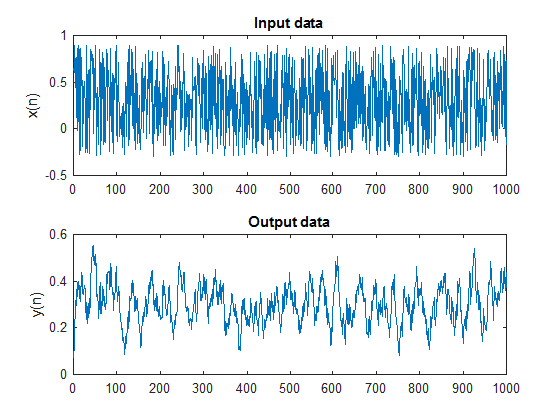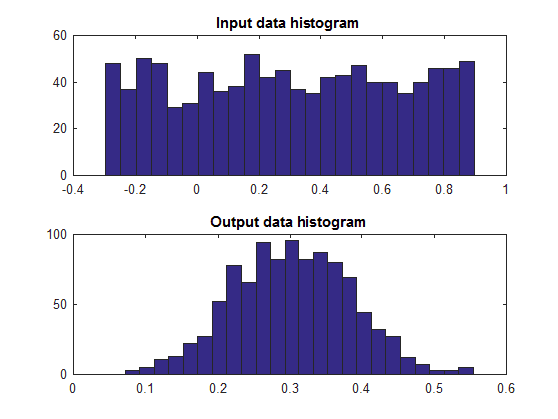And this is result with convolution formula: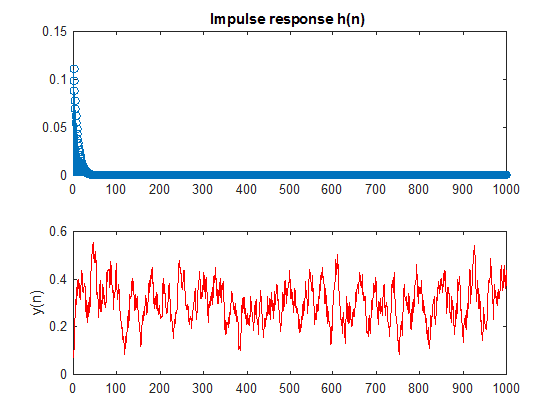There I tested that I can get same results with convolution formula and orginal filter formula. Is there all OK?

But I guess that is not what I exactly need to explain output distribution. As I understand your hints, I need to do convolution operation with two different distribution functions.
I know probability density function for uniform distribution (input data):
$$F(x) =\frac {x-a+1} {b-a+1}, x\in [a, b]$$
But it's not clear with "weighted, previous distribution function of the output". How can I describe it with probability density function?

Homework Helper
Gold Member
I like your approach to this question. I hadn't thought, that output is sum of two different distributions. Actually output isn't Gaussian distribution, but it just looks like that?
Right. If my intuition and memory serves me, the probability density function of the filter's output will not be Gaussian unless $T$ approaches infinity. (Don't ask me to prove that though.)

In this exercise, $T$ is 9. The number 9 is pretty close to infinity, which is why the output looks sort of Gaussian, but it's not quite there, so the distribution function is not truly Gaussian.

By the way, if the probability density function of the input was Gaussian, then the filter's output would be Gaussian regardless of what $T$ is. But that's a special case that does not apply to this exercise.
Filter output can be calculated recursively using difference equation:
$$y(n) = \sum_{k=0}^M b_nx(n-k)+\sum_{k=1}^N a_ky(n-k)$$
Or using convolution operation:
$$y(n) =h(n) * x(n),$$
where ##h(n)## is impulse response.
In my case transfer function of a IIR discrete time filter:
$$H(z) =\frac {0.111} {1-0.889z^{-1}}$$

So then I tested few things in Matlab:
Code:
clear all, clc
n=1000;
x = -0.3 + (0.9-(-0.3)).*rand(n,1);
y = filter(0.111,[1 -0.889],x);
figure(1)
subplot(211)
plot(x)
title('Input data')
ylabel('x(n)')
subplot(212)
plot(y)
title('Output data')
ylabel('y(n)')
figure(2)
subplot(211)
hist(x,24)
title('Input data histogram')
subplot(212)
hist(y,24)
title('Output data histogram')
figure(3)
a=[1, -0.889];
b=[0.111];
[h,t]=impz(b,a,1000);
subplot(211)
stem(t,h);
title('Impulse response h(n)')
subplot(212)
y1=conv(h,x)
plot(y,'-r')
ylabel('y(n)')

And this is result with convolution formula:
There I tested that I can get same results with convolution formula and orginal filter formula. Is there all OK?
Yes, I think that looks right to me.Except if I were you I would change your 0.111 and 0.889 numbers to 1/9 and 8/9 (or at least drag out the precision a little longer). Filters, whether they be analog or digital (including discrete time filters), can in some cases be pretty sensitive to precision and rounding errors.

Of course, filters involve convolution as part of their very operation.

But the process for finding the mathematically precise probability density function (pdf) of a filter uses a different process than what the filter uses directly, even though they both involve convolution.

Both processes use convolution. But they use it in different ways. More on this below.

But I guess that is not what I exactly need to explain output distribution. As I understand your hints, I need to do convolution operation with two different distribution functions.
Yes, the process involves convolving two different probability density functions. And more so, it involves repeating this process a large (perhaps approaching infinity) number of times.
I know probability density function for uniform distribution (input data):
$$F(x) =\frac {x-a+1} {b-a+1}, x\in [a, b]$$
Firstly, that's closer to the Cumulative Probability Function, not the Probability Density function.

Secondly, you don't need the '1's. I suggest getting rid of those.

I would say the cumulative probability function $F_x (x)$, for a uniform random variable is
$$F(x) = \begin{cases} 0 & x < a \\ \frac{x - a}{b - a} & a \leq x \leq b \\ 1 & x > b \end{cases}$$

To find the Probability Density Function (PDF), just take the derivative of that. It should give you as constant, non-zero value between $a$ and $b$ and 0 elsewhere.

So the probability density function $f_x$ is
$$f_x = \begin{cases} 0 & x < a \\ \frac{1}{b - a} & a \leq x \leq b \\ 0 & x > b \end{cases}$$

But it's not clear with "weighted, previous distribution function of the output". How can I describe it with probability density function?

I'll try to get you started.

Suppose we had a random variable what was uniformly random between 0 and 1. Let's call that variable $x$. Let's call its PDF $f_x$.

Since the function $f_x$ is a probability density function, the area under the curve must be 1. So in this case, the amplitude of $f_x$ is 1 between $0 \leq x \leq 1$ and zero everywhere else.

Probability density function for our example $f_x$:
$$f_x = \begin{cases} 0 & x < 0 \\ 1 & 0 \leq x \leq 1 \\ 0 & x > 1 \end{cases}$$

So what if we were to multiply $x$ by $\frac{1}{10}$. What is the probability density function, $f_{x/10}$, of that?

If you were to guess that it would be $\frac{1}{10} f_x$ that would seem deceptively simple. And it would also be wrong. The maximum possible value for $x$ is 1. So the maximum possible value for $\frac{1}{10}x$ is $\frac{1}{10}$. And we also need to scale the amplitude by the inverse of our constant so that the area under the curve remains 1.

Probability density function for our example $f_{x/10}$:
$$f_{x/10} = \begin{cases} 0 & x < 0 \\ 10 & 0 \leq x \leq 0.1 \\ 0 & x > 0.1 \end{cases}$$

So when we multiply our random variable by a small fraction, the resulting probability density function gets squished up; it gets narrower. But the height of the function grows taller (keeping the area under the curve a constant 1).

---

This is the sort of thing you will have to do in your problem. The input signal gets multiplied by $1/9$. So you'll have to find a new probability density function of the input $x$ that has a PDF that is squished to 1/9th and 9 times taller.

You'll then convolve that result with the PDF of the filter's previous output, scaled by $8/9$

----

It might help to start from the beginning. $u_o = x_0$, so the PDF of $u_0$ has the same PDF as the input.

To find the PDF of $u_1$ you'll need to convolve $f_{x/9}$ with $f_{8 u_0/9}$

Note that the PDF of $u_1$ is not uniform. It is certainly not Gaussian either. From here on out, you'll need to treat the PDFs of the outputs as having arbitrary shapes since they're neither Gaussian nor uniform, but somethings sort of in between (sort of).

Keep on going indefinitely with the pattern.And good luck!

Last edited:
•evol_w10lv
Homework Helper
Gold Member
Oh, and by the way, even though you are working with a discrete time filter, the PDFs involved are continuous functions (unless you are artificially imposing digital constrains such as those associated with a 32-bit floating point value or some such. But I don't think that is what you are interested in). The convolutions that I was talking about in my last post were continuous convolutions, not discrete.

So when you calculate the output stream by convolving the input stream with $h[n]$, then that is discrete convolution. That's fine. That's what discrete time filters do. But that doesn't tell you anything about the probability distribution of the output.

When you are finding the resulting PDFs, calculating $f_{u[n]} = f_{8 u[n-1]/9} \ast f_{x/9}$, that is continuous convolution to be done with integration.

Last edited:
•evol_w10lv
evol_w10lv
Input distribution:
$$f_x = \begin{cases} 0, & x \lt -0.3 \\ \frac {1} {1.2}, & -0.3 \leq x \leq 0.9 \\ 0, & x \gt 0.9 \end{cases}$$
Input distribution scaled by ##1/9##::
$$f_{x/9} = \begin{cases} 0, & x \lt -\frac {1} {30} \\ 7.5, & -\frac {1} {30} \leq x \leq 0.1 \\ 0, & x \gt 0.1 \end{cases}$$
Filter's previous output scaled by ##8/9##:
$$f_{8u_{0}/9} = \begin{cases} 0, & x \lt -\frac {4} {135} \\ 8\frac {7} {16}, & -\frac {4} {135} \leq x \leq \frac {4} {45} \\ 0, & x \gt \frac {4} {45} \end{cases}$$
Then I need to calculate:
$$f_{u_{n}}(z) = \int_{\infty}^{-\infty} f_{8u_{n-1}/9}(x) f_{x/9}(z-x) \, dx$$
First iteration n=1:
$$f_{u_{1}}(z) = \int_{\infty}^{-\infty} f_{8u_{0}/9}(x) f_{x/9}(z-x) \, dx$$

But I have got 1000 random numbers (input), is it mean that to calculate resulting PDF I need to do n=1000 iterations?Homework Helper
Gold Member
Input distribution:
$$f_x = \begin{cases} 0, & x \lt -0.3 \\ \frac {1} {1.2}, & -0.3 \leq x \leq 0.9 \\ 0, & x \gt 0.9 \end{cases}$$
Input distribution scaled by ##1/9##::
$$f_{x/9} = \begin{cases} 0, & x \lt -\frac {1} {30} \\ 7.5, & -\frac {1} {30} \leq x \leq 0.1 \\ 0, & x \gt 0.1 \end{cases}$$
Filter's previous output scaled by ##8/9##:
$$f_{8u_{0}/9} = \begin{cases} 0, & x \lt -\frac {4} {135} \\ 8\frac {7} {16}, & -\frac {4} {135} \leq x \leq \frac {4} {45} \\ 0, & x \gt \frac {4} {45} \end{cases}$$
Then I need to calculate:
$$f_{u_{n}}(z) = \int_{\infty}^{-\infty} f_{8u_{n-1}/9}(x) f_{x/9}(z-x) \, dx$$
First iteration n=1:
$$f_{u_{1}}(z) = \int_{\infty}^{-\infty} f_{8u_{0}/9}(x) f_{x/9}(z-x) \, dx$$

But I have got 1000 random numbers (input), is it mean that to calculate resulting PDF I need to do n=1000 iterations?Good work so far!Before we go on though, I must ask: were you really tasked with finding the actual (mathematically exact) probability density function?

In your original post, the question was asking for a reason why the filter's output's probability density function looked Gaussian (or at least Gaussian-like) when the input's probability density function was uniform.

I ask because I think you are very close to being able to answer
1. why the filter's output's probability density function goes from being uniform to one that looks sort-of Gaussian like, and
2. why the filter's output's probability density function will never be actually Gaussian, even if it is sort of close.
On the other hand, solving for the (mathematically exact) probability density function is a lot tougher. (Heck, I'm not even sure I'm up for that challenge.)

---

You might want to try going through a few iterations to see what happens to $f_{u_n}$ at each iteration. I think that will help show 1) above, where the function starts out as uniform, then starts to look more Gaussian (sort of) with each iteration. (More on the second answer later.)

The following might help with the second answer, and if you actually did wish to solve for the actual function.

If we wanted to scale a random variable by some amount, say by $\frac{8}{9}$, and we know the original probability density function, say we call it $f_{u_n}(x)$, then the probability density function of the scaled random variable is
$$f_{8u_n/9}(x) = \frac{9}{8}f_{u_n}(9x/8)$$
Make sure that makes sense to you before we move on. (And take note for later [see below] that if the original function is Gaussian, the density function of the scaled random variable is also Gaussian, albeit with a different mean and standard deviation.)

After an infinite number of iterations (as $n \rightarrow \infty$), the probability density function is not going to change anymore. In other words, $f_{8u_n/9}(x) = f_{8u_{(n-1)}/9}(x)$.

And with that bit of insight, we can say,
$$f_{u_n}(x) = \int_{-\infty}^\infty \frac{9}{8}f_{u_n}(9z/8) f_{x/9}(x-z)dz$$
And that's the differential equation that will ultimately give you your answer. Notice that there is only one function that is unknown, and that is $f_{u_n}()$. You already know what $f_{x/9}()$ is, you've calculated that above (I did some variable substitution, btw).

What that differential equation is asking is as follows: "Suppose I had an original function and then scaled it a little bit, in a known way, to make a new function, then I convolved that with this other, known function such that the result is equal to the original function. What [original] function meets these requirements?"

I'm confident that the differential equation has a solution. But I'm not confident that I have the mathematical aptitude to solve for it. (Nor am I positive that it can be represented in closed form.) [Edit: I just came up with an idea or two on how this differential equation might be solved. But I haven't worked out the details yet.]

Even without solving for the actual function, you might be able to convince yourself why the solution is not Gaussian. Is it possible to convolve a Gaussian function with one and only one other non-Gaussian function* and produce a truly Gaussian function?

*[Edit: and not something trivial like an impulse either. I mean convolved with a uniform function, for example.]

Last edited:
•evol_w10lv
evol_w10lv
were you really tasked with finding the actual (mathematically exact) probability density function?
Actually I think that I don't need mathematically exact probability density function, that's why I didn't ask for it. Heh, we started to speak about probability density functions, then I thought that I could solve actual function. But I think I won't be able to solve that continuous convolvution integral.

So I will try to explaine the question in my homework without mathematically exact output probability density function.

By the way, I can't get it one thing:
$$f_{8u_{n}/9}(x)=\frac {9}{8}f_{u_{n}}(9x/8)$$
Why and how scale factor ##8/9## started to react like ##9/8## in the second part of equation?

When I got the answer whether my explanation is OK or not, I will inform you here.

Homework Helper
Gold Member
By the way, I can't get it one thing:
$$f_{8u_{n}/9}(x)=\frac {9}{8}f_{u_{n}}(9x/8)$$
Why and how scale factor ##8/9## started to react like ##9/8## in the second part of equation?

When I got the answer whether my explanation is OK or not, I will inform you here.

Multiplying the function's input by a number greater than 1 (i.e., the $\frac{9}{8}$ in $f(9 x /8)$) squishes the function along the x-axis. With this scaling factor, the output of the function when $x = \frac{8}{9}$ produces the same output as the original function when $x = 1$.

Scaling the output of the function by the same factor ensures that the area under the curve remains 1.

You have already calculated the relevant functions, $f_x(x)$, $f_{x/9}(x)$, and $f_{8x/9}(x)$ back in post #7. As a sanity check, go back and plug some numbers into them and ensure that
$$f_{x/9}(x) = 9 f_x(9x)$$
and
$$f_{8x/9}(x) = \frac{9}{8} f_x(9x/8) .$$

As an additional sanity check you should be able to confirm that the area under each curve is equal to 1.

[Edit: regarding my above notation, recall that $u_0 = x_0$, thus $f_{8u_0/9}(x) = f_{8x/9}(x)$. As far as the differential equation goes though, we don't know what $f_{u_n}(x)$ is as $n \rightarrow \infty$, yet. That is the unknown function for which the differential equation is trying to solve (and we haven't solved that yet). But we do know, whatever that function is, that $f_{8u_n/9}(x) = \frac{9}{8} f_{u_n}(9x/8)$.]

Last edited:
•evol_w10lv
evol_w10lv
Now I understand that part. Thanks.
I think I calculated wrong ##f_{8u_{0}/9}## in post #7. It should be ##f_{x}## scaled by ##8/9##, because ##x_{1}=u_{0}##. But I did something else there.

You might want to try going through a few iterations to see what happens to ##f_{u_n}## at each iteration. I think that will help show 1) above, where the function starts out as uniform, then starts to look more Gaussian (sort of) with each iteration.

Then I tried to solve few discrete convolution iterations in Matlab. Here are results: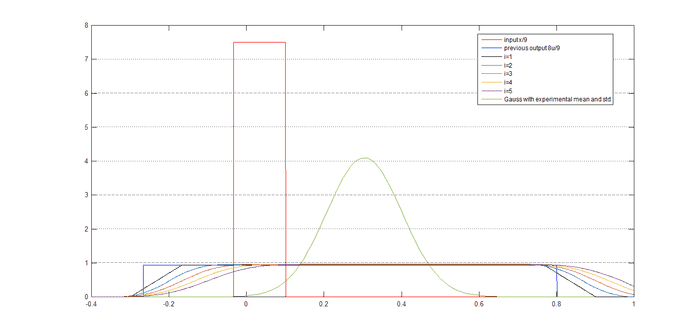Something looks incorrect there.. Of course, I can see how from two uniform distributions, sum of distributions starts to look like Gaussian. But values seems incorrect.
Green line is Gaussian pdf with experimental mean and std from here (output data histogram):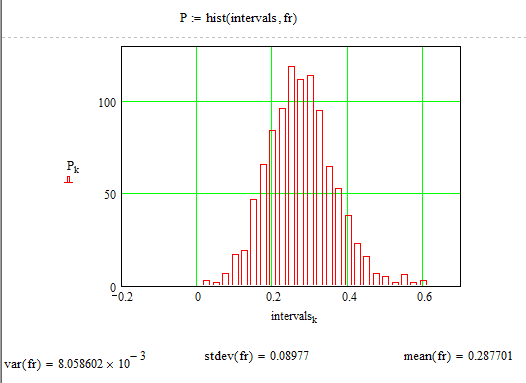I think (and actually you said it) when n goes to infinity (or even as it is in my case n = 1000), my output pdf should be simular like that Gaussian pdf, but in my case amplitude is about value 1, which is not close to Gaussian max amplitude value (4). I guess, ##f_{8u_{0}/9}## is wrong.
It's late now, I will chek it tomorrow one more time. And I think it will be OK without mathematically exact output probability density function to prove output. Graphic with iterations shows very well how convolution works and how sum of distributions starts to look like Gaussian. Just need to correct values there, I guess.

evol_w10lv
I went through my calculations.
Input distribution:
$$f_x = \begin{cases} 0, & x \lt -0.3 \\ \frac {1} {1.2}, & -0.3 \leq x \leq 0.9 \\ 0, & x \gt 0.9 \end{cases}$$

Input distribution scaled by ##1/9##::
$$f_{x/9} = \begin{cases} 0, & x \lt -\frac {1} {30} \\ 7.5, & -\frac {1} {30} \leq x \leq 0.1 \\ 0, & x \gt 0.1 \end{cases}$$

In the first iteration is filter's previous output scaled by ##8/9##, which actually is ##f_{x}## scaled by ##8/9##:
$$f_{8u_{0}/9} = \begin{cases} 0, & x \lt -\frac {4} {15} \\ \frac {15} {16}, & -\frac {4} {15} \leq x \leq 0.8 \\ 0, & x \gt 0.8 \end{cases}$$

Matlab code:
Code:
clear all, clc
T=0.001;
t=-0.267:T:0.801;
t2=-0.267*2:T:0.801*2
x1=7.5*(t>-1/30) - 7.5*(t>0.1);
x11=7.5*(t2>-1/30) - 7.5*(t2>0.1);
u0=0.9375*(t>-4/15) - 0.9375*(t>0.8);
u1=T*conv(u0,x1);
figure(1)
plot(t,x1,'red',t,u0,'blue')
hold on
plot((-0.267*2):T:(0.801*2),u1,'green')
grid on
xlim([-0.4 1])
legend('pdf x1','pdf u0','pdf u1=x1*u0')

u2=T*conv(u1,x11);
figure(2)
plot(t2,x11,'red',t2,u1,'blue')
hold on
plot((-0.267*2*2):T:(0.801*2*2),u2,'green')
grid on
xlim([-0.4 1])
legend('pdf x1','pdf u1','pdf u2=x1*u1')

n=1
##f_{u} = f_{8 u/9} \ast f_{x/9}##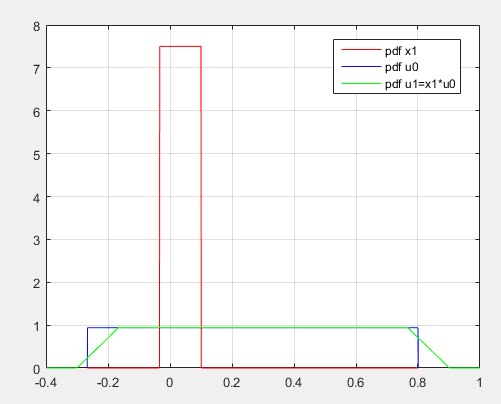n=2
##f_{u} = f_{8 u/9} \ast f_{x/9}##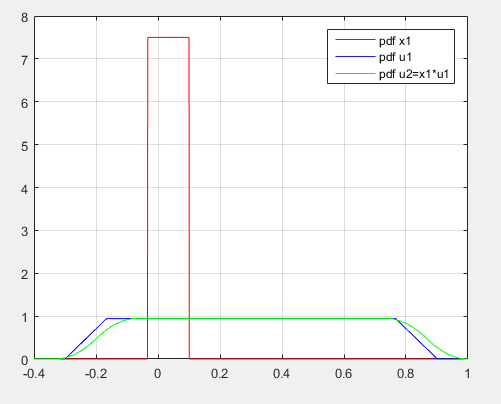And now, writing this post, I realized where's my mistake.. I didn't scale ##f_{u}##. I used ##f_{u}## in n=2, but I need ##f_{8 u/9}##. Ehhh..

Last edited: